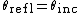The returning or throwing back of electromagnetic radiation such as light, ultraviolet rays, radio waves, or microwaves by a surface upon which the radiation is incident. In general, a reflecting surface is the boundary between two materials of different electromagnetic properties, such as the boundary between air and glass, air and water, or air and metal. Devices designed to reflect radiation are called reflectors or mirrors.

The simplest reflection laws are those that govern plane waves of radiation. The law of reflection concerns the incident and reflected rays (as in the case of a beam from a flashlight striking a mirror) or, more precisely, the wave normals of the incident and reflected waves. The law states that the incident and reflected rays and the normal to the reflecting surface all lie in one plane, called the plane of incidence, and that the reflection angle equals the angle of incidence as in

(1)Eq. (1) [see illustration]. The angles Θinc and Θrefl are measured between the surface normal and the incident and reflected rays, respectively. The surface (in the above example, that of the mirror) is assumed to be smooth, with surface irregularities small compared to the wavelength of the radiation. This results in so-called specular reflection. In contrast, when the surface is rough, the reflection is diffuse. An example of this is the diffuse scattering of light from a screen or from a white wall where light is returned through a whole range of different angles.

The reflectivity of a surface is a measure of the amount of reflected radiation. It is defined as the ratio of the intensities of the reflected and incident radiation. The reflectivity depends on the angle of incidence, the polarization of the radiation, and the electromagnetic properties of the materials forming the boundary surface. These properties usually change with the wavelength of the radiation. Reflecting materials are divided into two groups: transparent materials also called dielectrics, and opaque conducting materials, usually metals.

The reflectivity of polished metal surfaces is usually quite high. Silver and aluminum, for example, reflect more than 90% of visible light. In ordinary mirrors the reflecting surface is the interface between metal and glass, which is thus protected from oxidation, dirt, and other forms of deterioration. When it is not permissible to use this protection for technical reasons, one uses “front-surface” mirrors, which are usually coated with evaporated aluminum.

The material property that determines the amount of radiation reflected from an interface between two dielectric media is the phase velocity v of the electromagnetic radiation in the two materials. In optics one uses as a measure for this velocity the refractive index n of the material, which is defined

(2)by Eq. (2) as the ratio of the velocity of light c in vacuum and the phase velocity in the material. For visible light, for example, the refractive index of air is about n = 1, the index of water is about n = 1.33, and the index of glass is about n = 1.5. See Phase velocity, Refraction of waves

For normal incidence (Θinc = 0) the reflectivity R of the interface is given by

(3)Eq. (3), in which the material constants are labeled 1 and 2, where the radiation is incident in material 1. The reflectivity of an air-water interface is about 2% (R = 0.02) and that of an air-glass interface about 4% (R = 0.04); the other 98% or 96% are transmitted through the water or glass, respectively. See Geometrical optics, Mirror optics, Reflection of sound

McGraw-Hill Concise Encyclopedia of Physics. © 2002 by The McGraw-Hill Companies, Inc.
Site: Follow: Share:
Open / Close